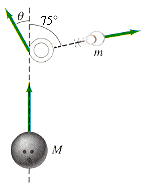# Three dimensional collision with bowling ball and pin.

## Homework Statement

In order to convert a tough split in bowling, it is necessary to strike the pin a glancing blow as shown in the figure. Assume that the bowling ball, initially traveling at 15.0 m/s, has eight times the mass of a pin and that the pin goes off at 75° from the original direction of the ball.(a) Calculate the speed of the pin.
(b) Calculate the speed of the ball just after collision.
(c) Calculate the angle θ through which the ball was deflected. Assume the collision is elastic and ignore any spin of the ball.

## Homework Equations

MbVb+MpVp=MbVB+MpVP (Mb=mass of ball, Mp=mass of pin, Vp=initial velocity for pin, VP=final velocity for pin, Vb=initial velocity for ball, VB=final velocity for ball.)

## The Attempt at a Solution

I have no idea how to even attempt this problem.

## The Attempt at a Solution

Try writing down your Conservation of Momentum equations

Should I write down the formula first? Should I plug in the numbers and continue trying to cancel out unknown variables? The conservation of energy and momentum equations are realllllly long -_-

I would like too bump this-I have the same question!
I tried so far using the p=mv equation in both dimensions (since in the x direction it equals zero!), however I can't seem to solve for what they're asking…my algebraic manipulations aren't as well.

CWatters
Homework Helper
Gold Member
This is in the homework section and the forum requires you to show your working/attempt. How else can we see where you are going wrong ;-)

Should I write down the formula first? Should I plug in the numbers and continue trying to cancel out unknown variables? The conservation of energy and momentum equations are realllllly long -_-

It's not usually a good ide to plug in the numbers early on.

bobie
Gold Member
the bowling ball, initially traveling at 15.0 m/s, has eight times the mass of a pin and that the pin goes off at 75° from the original direction of the ball.
Hi, I do not know if the formula for balls is valid for pins, if it is ...then
you know cos λp (75°) = 0.2588 and the ratio Rm of Mb to total mass 8/9
you get quickly vp = v0* cos λ* 2R (15*0.25*16/9) = 6.9 m/s
the you can easily find all missing data (with the E and p formulas.)

CWatters